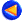### SEMINAIRE D'ANALYSE NUMERIQUE Année universitaire 2013-2014

Jeudi 5 juin 2014 :   (Universita di Padova)
Eikonal equation on the Sierpinski gasket. Résumé.

We study the eikonal equation on the Sierpinski gasket following the model of the Laplacian. On prefractals, we consider graph and network eikonal equations; we show that the solutions of these problems converge to a function defined on the fractal set. We characterize this limit function as the unique "metric" viscosity solution to the eikonal equation on the Sierpinski gasket according to the definition introduced in a recent paper by Giga, Hamamuki and Nakayasu.

Joint work with: F. Camilli (univ. Roma) and R. Capitanelli (Univ. Roma).